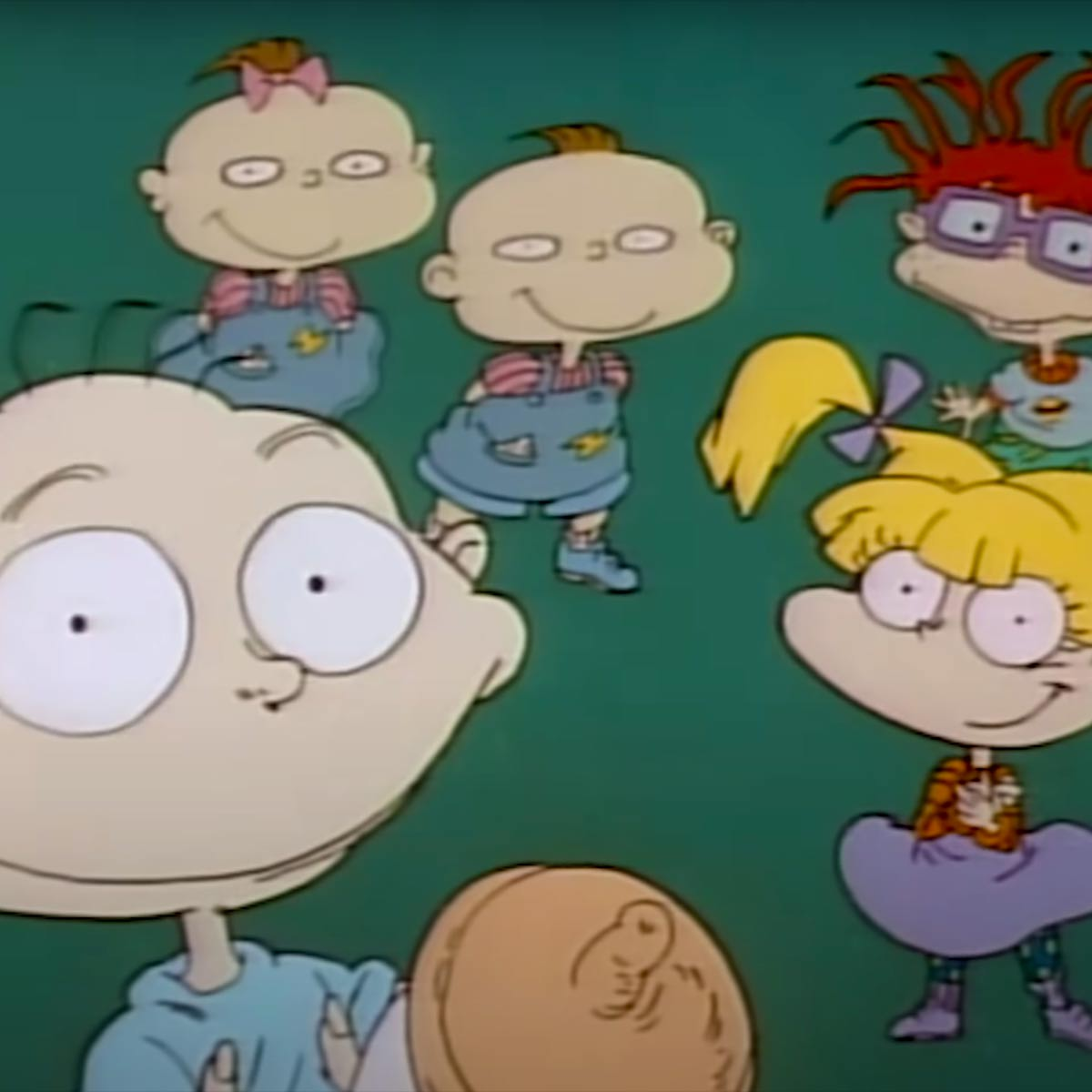# Rugrats (Theme)

10 September 2020
5
00:26
• ##### TEMPO
100
0
(0)

8 [ut] [yi] [uo] [pi4] [oa] [sp]
5 [oa]| [uo] [pi4] [uo] [yi]
8 [ut] [yi] [uo] [pi4] [oa] [sp]
5 [da]| [oa] [sp4] [oa] [pi]
8 [ut] [yi] [uo] [pi4] [oa] [sp]
5 [oa]| [uo] [pi4] [uo] [yi]
8 [ut] [yi] [uo] [pi4] [oa] [sp]
[da5] [sp] [oa] [pi4] [uo] [yi]
[ut8]| |5||[ya] [us8]

Rate This Music Sheet:

Average rating 0 / 5. Vote count: 0

No votes so far! Be the first to rate this music sheet.

Thank you for rating this song!

If you have any specific feedback about how to improve this music sheet, please submit this in the box below.

Rugrats (Theme) is a song by Mark Mothersbaugh. Use your computer keyboard to play Rugrats (Theme) music sheet on Virtual Piano. This is an Intermediate song and requires a lot of practice to play well. The recommended time to play this music sheet is 00:26, as verified by Virtual Piano legend, Mark Chaimbers. The song Rugrats (Theme) is classified in the genres: Songs From TV, Kids on Virtual Piano. You can also find other similar songs using 90s Songs, Fun.

## Related

•[C5] k x k z k kxCn| |[x\$] j z j L j jxCb| |[C5] k x k z k Gjkx| |[x\$] j z j L j afGj| |[yo0]|p|a|[upQ]|a|S|[waI]|S|d|[pQ] T u I p S G|j| |a p [wa]|[yrI]|G|[SQ]|[ueT] d S p [a0]|[ywr] S d f [j9] k [jeTQ]| |[wa]|[yrI]|G|[jQ]|[ueTS]|p|[ra]|[uaI] p S|[ra]|[aYI]| |G|j|k| |j k j| |f|G|j|S|d| |d f G||G f G f| |d f G|h|j|J|k|L|k| |j k j| |f|G|j|L|z|x|C|C| |x z L| |j L z||z L k J|k|L|C| |x z L|j|G|k| |a|p|[a5]|[Q97]|G|[S\$]| d S p [a3]| S d f [j2] k [j6\$]| |[a5]|[Q97]|G|[j\$]|[S60*]|p|[a7]|[raQ0] p S|[a7]|[raQ(]| |G|j|[wk]|[yrI]|j k [je]|[uTI]|f|[QG]|[ujeT]|S|[dQ]|[yrI]|d f [G0]|[ywr] G f G [fQ]|[ueT]|d f [wG]|[yrhI]|j|[QJ]|[kTIE]|L|[wk]|[yrI]|j k [je]|[uTI]|f|[QG]|[ujeT]|L|[zQ]|[yxrI]|C|[C0]|[ywr]|x z [QL]|[ueT]|j L [zw]|[yrI] z L k [QJ]|[ukIE]|L|[wC]|[yrI]|x z [QL]|[ujeT]|G|[rk]|[uaI]| |r|[yaI]| |[oC]| |x z [pL]|b|C|[na]

Level: 6
Length: 02:54
Intermediate

#### Bruno Wen-li

•[tqi] s s p p|p| [ye9] p p o p g f| [upe] f f s s|s| [ywo] d d a a|a| [q84] t [to] [pe] [se]|[fe]| [d962] e e w e i u| [e60] t [tp] e [se]|e| [wf95] [yd] [ys] [ra] r p [ro]| [q84] t [to] [pe] [se]|[fe]| [d962] e e w e i u| [e60] u [up] t [ts]|t| [wf95] [yd] [ys] [ra] r p [ro]| [q84] t [tj] e [he]|[fe]| [d962] e e w e i u| [e60] u [uj] t [th]|[tf]| [wd95] [yd] [yf] [rd] r s [rp]| [q84] t [to] [pe] [se]|[ea]| [a962] [pe] [oe] w e i [uo] p [se60] [us] [up] t [ts] spt p [ws95]s[ys]s[yp] [rp] [sr] spr d [sq84] s s p p|p|  p p o p g f| [ed60] d d f d s s| [wd95] d d f d s s| [qp84] s s p p|p|  p p o p g f| [ed60] d d f d s s| [wd95] d f d s|h
Level: 6
Length: 00:55
Intermediate

#### Calvin Harris

•[0u] r I r o r a r
[8u] t I t o t a t
[6u] T I T o T a T
[7Y] r u r I
[3u] r I r o r a r
[1u] t I t o t a t
[6u] T I T o T a s [7a] [0r] f [8wd] s [60a] [0r] f [G8w] h [f60] [0r] f [8wd] s [a60] a s [aw8] s a s a s a o [IQ7] [0r] f [8wd] s [60a] [0r] a f
[G8w] h [f60] [0r] f [d8w] s [a60] s [a8w] s a s a s a o [IQ7] I o [pw8] o I [o8w] u I o [pw8] o I [o8w] I o [p0e] o o p [a0e] s a s [a7Q] a h [G0r] a [fw8] s [a06] a h [G0r] f [hw8] k j [f06] k l [k0r] h j [fw8] k l [k0r] h j [fw8] k l [k06] j z l k h j [kr0] h
[G8w] d [f06] h G h G h [fr0] G h k [fw8] G h k [60f] G h k [DQ7] f G [0rf] [dw8] s [a60] p [0r] d f [Gw8] f G h [f60] [0r] d f [dw8] s a s
[a60] p a s [aw8] s a f [GQ7] a h [G0r] a [fw8] s [a06] a h
[G0r] f [hw8] k [j06] f k l [k0r] h j [fw8] k l [k06] j z l k h j
[k0r] h [Gw8] d f h G h G h [fr0] G h k [fw8] G h k [f06] G h k [DQ7] f G [fr0] G h k [fw8] G h k [f06] G h k [DQ7] f G k l [ku] h j [ft] k l [ke] h h G f h j [ku] h [Gt] d [fe] h G h G h f [fr0]

Level: 5
Intermediate

#### Billie Eilish